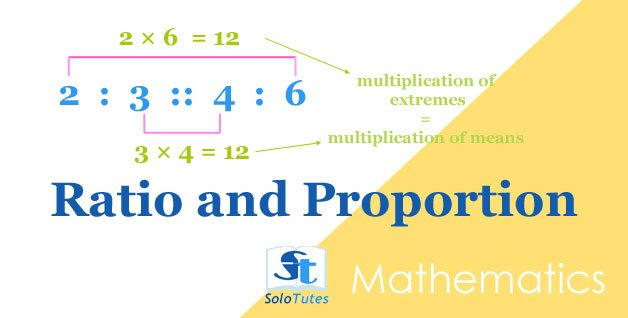SoloTutes

2 Posts
Followers ×## Mathematics: Profit And Loss, Solved Questions

The profit earned by selling an article for Rs 900 is double the loss incurred when the same article is sold for Rs 450. At what price should the## Ratio and Proportion, Basic Tricks and Practice Problems

ratio  can be written in the form of a :b ( where a and b are integers) is the simplified fractional relation ( a/b)  between two quan

### Do you Want to Write Academic Notes Here?

Join SoloTutes.com Telegram Channels@pharmacy_exams@nurseexams@solotutes